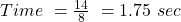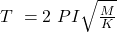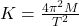## A mass of 50.00g hangs from a 7.00cm-long spring that is rigidly attached to a ceiling. The mass is pulled down slightly, let go, and is obs

Question

A mass of 50.00g hangs from a 7.00cm-long spring that is rigidly attached to a ceiling. The mass is pulled down slightly, let go, and is observed to make 8 round trips (up and back down) in 14.00s. What is the stiffness constant for this spring

in progress 0
2 months 2021-08-23T23:10:47+00:00 1 Answers 3 views 0

0.645 N/M

Explanation:

Given

Mass=50.00g

We have to convert into the kg

So Mass =0.050 KgWe know that……………………Eq(1)

Where T= time

and M= Mass

K= Stiffness constant

On squaring both side we get….Eq(2)

Putting the value of M ,T and π in Eq(2) we get

K=0.645 N/M